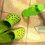# 75^40=9^20*5^x

can anyone tell me how to solve this.Note by Daniel Kua
6 years, 5 months ago

This discussion board is a place to discuss our Daily Challenges and the math and science related to those challenges. Explanations are more than just a solution — they should explain the steps and thinking strategies that you used to obtain the solution. Comments should further the discussion of math and science.

When posting on Brilliant:

• Use the emojis to react to an explanation, whether you're congratulating a job well done , or just really confused .
• Ask specific questions about the challenge or the steps in somebody's explanation. Well-posed questions can add a lot to the discussion, but posting "I don't understand!" doesn't help anyone.
• Try to contribute something new to the discussion, whether it is an extension, generalization or other idea related to the challenge.
• Stay on topic — we're all here to learn more about math and science, not to hear about your favorite get-rich-quick scheme or current world events.

MarkdownAppears as
*italics* or _italics_ italics
**bold** or __bold__ bold
- bulleted- list
• bulleted
• list
1. numbered2. list
1. numbered
2. list
Note: you must add a full line of space before and after lists for them to show up correctly
paragraph 1paragraph 2

paragraph 1

paragraph 2

[example link](https://brilliant.org)example link
> This is a quote
This is a quote
    # I indented these lines
# 4 spaces, and now they show
# up as a code block.

print "hello world"
# I indented these lines
# 4 spaces, and now they show
# up as a code block.

print "hello world"
MathAppears as
Remember to wrap math in $$ ... $$ or $ ... $ to ensure proper formatting.
2 \times 3 $2 \times 3$
2^{34} $2^{34}$
a_{i-1} $a_{i-1}$
\frac{2}{3} $\frac{2}{3}$
\sqrt{2} $\sqrt{2}$
\sum_{i=1}^3 $\sum_{i=1}^3$
\sin \theta $\sin \theta$
\boxed{123} $\boxed{123}$

## Comments

Sort by:

Top Newest

Which one do you mean I or II :

• $75^{40} = 9^{20 \times 5^{x}} . . . . . I$

• $75^{40} = 9^{20} \times 5^{x} . . . . .II$

- 6 years, 5 months ago

Log in to reply

no.2

- 6 years, 5 months ago

Log in to reply

how do u type multiple again? tell me pls.

- 6 years, 5 months ago

Log in to reply

You can see how to type in math formatting by click on "formatting guide" at the bottom-left corner of your comment box.

To type multiple, just type " \times " in math format (remember, in math format! See how to start a math format in the formatting guide.

The more easy ways to know the format of symbols, just point your cursor at the symbols, the format then will appears.

- 6 years, 5 months ago

Log in to reply

the 2nd one

- 6 years, 5 months ago

Log in to reply

Sorry for the late response,

$75^{40} = 9^{20} \times 5^{x}$

$75^{40} = (3^2)^{20} \times 5^{x}$

$75^{40} = 3^{40} \times 5^{x}$

$\frac{75^{40}}{3^{40}} = 5^{x}$

$(\frac{75}{3})^{40} = 5^{x}$

$25^{40} = 5^{x}$

$(5^2)^{40} = 5^{x}$

$5^{80} = 5^{x}$

$\boxed{x = 80}$

- 6 years, 5 months ago

Log in to reply

$75^{40}= (3*25)^{40}$

$= 3^{40} * 25^{40}$

$= 3^{2*20} * (5^2)^{40}$

$= (3^2)^{20} * 5^{2*40}$

$= 9^{20}*5^{80}$

Therefore $x = 80$. I only used 2 formulae, $(ab)^n = a^n * b^n$ and $(a^n)^m = a^{m*n}$

Also, to write all the signs, read this

- 6 years, 5 months ago

Log in to reply

3^40 is not equal to 3^20+2

- 6 years, 4 months ago

Log in to reply

Of course not. Look at this exponent's formulas :

$a^x \times a^y = a^{x+y}$

$(a^x)^y = a^{xy}$

So,

$3^{40} \neq 3^{20+2}$ but

$3^{40} = 3^{2 \times 20} = (3^2)^{20}$

Got it?

- 6 years, 4 months ago

Log in to reply

Its $3^{20 \times 2}$. I used the $*$ symbol.

- 6 years, 4 months ago

Log in to reply

x=80

- 6 years, 4 months ago

Log in to reply

X=80

- 6 years, 2 months ago

Log in to reply

×

Problem Loading...

Note Loading...

Set Loading...